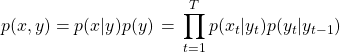## Suppose you are modeling text with a HMM, What is the complexity of finding most the probable sequence of tags or states from a sequence of text using brute force algorithm?

Assume there are total states and let be the length of the largest sequence. Think how we generate text using an hMM. We first have a state sequence and from each state we emit an output. From each state, any word out of possible outcomes can be generated. Since there are states, at each possible…

## How do you generate text using a hMM model given below:One possible interpretation of the latent variables in the HMM model is that they are POS tags. We will go with this interpretation for simplicity, though the latent states could mean other things as well. To generate text from a HMM, we need to know the transition matrix (the probability of going from one tag…